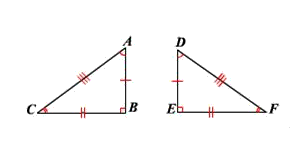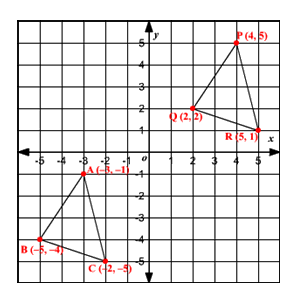# Congruent Triangles on the Coordinate Plane

Two triangles are said to be congruent if there is an isometry mapping one of the triangles to the other. (An isometry is a transformation , such as translation , rotation , or reflection , that doesn't change the distance between any two points.)

Imagine the two triangles are cut out of paper. If you can put one on top of the other and match its shape exactly, then the two triangles are congruent.In the above figure, $\Delta ABC$ is congruent to $\Delta DEF$ .

Recall the SSS Congruence Theorem: If three sides of one triangle are congruent to three sides of a second triangle, then the two triangles are congruent. Given two triangles on a coordinate plane, you can check whether they are congruent by using the distance formula to find the lengths of their sides. If three pairs of sides are congruent, then the triangles are congruent by the above theorem.

If $\stackrel{¯}{AB}\cong \stackrel{¯}{PQ}$ , $\stackrel{¯}{BC}\cong \stackrel{¯}{QR}$ , and $\stackrel{¯}{AC}\cong \stackrel{¯}{PR}$ , then $\Delta ABC\cong \Delta PQR$ .

Example:.

Verify that these triangles are congruent using the distance formula and the SSS Congruence Theorem.

Solution:

Find the side lengths using the Distance Formula.

$\text{Distance}=\sqrt{{\left({x}_{2}-{x}_{1}\right)}^{2}+{\left({y}_{2}-{y}_{1}\right)}^{2}}$

The triangle $ABC$ has the vertices $A\left(-3,-1\right)$ , $B\left(-5,-4\right)$ and $C\left(-2,-5\right)$ .

$\begin{array}{l}\stackrel{¯}{AB}=\sqrt{{\left(-5-\left(-3\right)\right)}^{2}+{\left(-4-\left(-1\right)\right)}^{2}}=\sqrt{13}\\ \stackrel{¯}{BC}=\sqrt{{\left(-2-\left(-5\right)\right)}^{2}+{\left(-5-\left(-4\right)\right)}^{2}}=\sqrt{10}\\ \stackrel{¯}{AC}=\sqrt{{\left(-2-\left(-3\right)\right)}^{2}+{\left(-5-\left(-1\right)\right)}^{2}}=\sqrt{17}\end{array}$

The triangle $PQR$ has the vertices $P\left(4,5\right)$ , $Q\left(2,2\right)$ and $R\left(5,1\right)$ .

Therefore,

$\begin{array}{l}\stackrel{¯}{PQ}=\sqrt{{\left(2-4\right)}^{2}+{\left(2-5\right)}^{2}}=\sqrt{13}\\ \stackrel{¯}{QR}=\sqrt{{\left(5-2\right)}^{2}+{\left(1-2\right)}^{2}}=\sqrt{10}\\ \stackrel{¯}{PR}=\sqrt{{\left(5-4\right)}^{2}+{\left(1-5\right)}^{2}}=\sqrt{17}\end{array}$

Here, the corresponding sides of the two triangles have the same lengths:

$\stackrel{¯}{AB}\cong \stackrel{¯}{PQ},\stackrel{¯}{BC}\cong \stackrel{¯}{QR}$ , and $\stackrel{¯}{AC}\cong \stackrel{¯}{PR}$

So, by the SSS Congruence Theorem, $\Delta ABC\cong \Delta PQR$ .